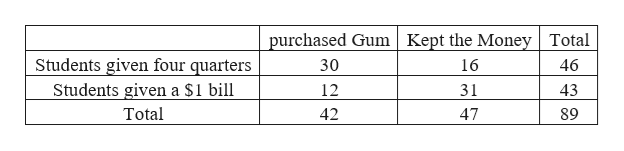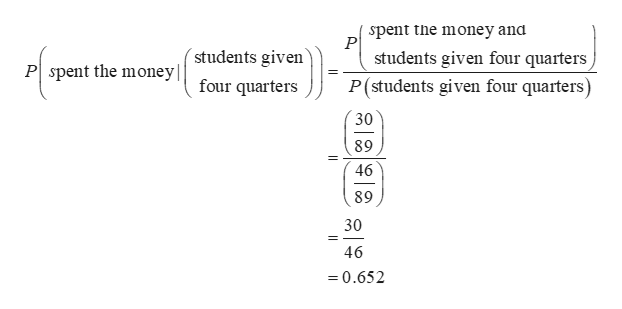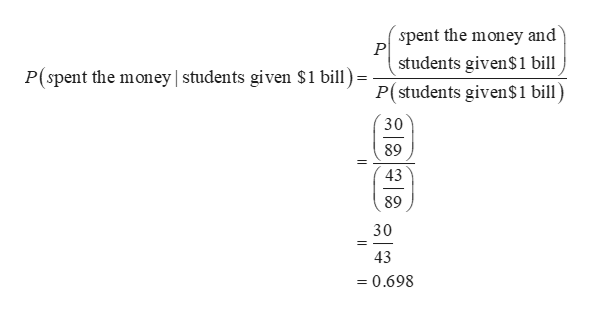# In an​ experiment, college students were given either four quarters or a​ \$1 bill and they could either keep the money or spend it on gum. The results are summarized in the table. Complete parts​ (a) through​ (c) below.  Purchased GumKept the Money Students Given Four Quarters3016 Students Given a​ \$1 Bill1231 a. Find the probability of randomly selecting a student who spent the​ money, given that the student was given four quarters. The probability is?​(Round to three decimal places as​ needed.)b. Find the probability of randomly selecting a student who spent the​ money, given that the student was given a​ \$1 bill. The probability is?​(Round to three decimal places as​ needed.)c. What do the preceding results​ suggest?  A.A student given a​ \$1 bill is more likely to have spent the money than a student given four quarters. B.A student was more likely to be given four quarters than a​ \$1 bill. C.A student given four quarters is more likely to have spent the money than a student given a​ \$1 bill. D.A student was more likely to have spent the money than to have kept the money.

Question
23 views
In an​ experiment, college students were given either four quarters or a​ \$1 bill and they could either keep the money or spend it on gum. The results are summarized in the table. Complete parts​ (a) through​ (c) below.

 Purchased Gum Kept the Money 30 16 12 31
a. Find the probability of randomly selecting a student who spent the​ money, given that the student was given four quarters.

The probability is?
​(Round to three decimal places as​ needed.)
b. Find the probability of randomly selecting a student who spent the​ money, given that the student was given a​ \$1 bill.

The probability is?
​(Round to three decimal places as​ needed.)
c. What do the preceding results​ suggest?

A.
A student given a​ \$1 bill is more likely to have spent the money than a student given four quarters.

B.
A student was more likely to be given four quarters than a​ \$1 bill.

C.
A student given four quarters is more likely to have spent the money than a student given a​ \$1 bill.

D.
A student was more likely to have spent the money than to have kept the money.
check_circle

Step 1

From the given information,help_outlineImage Transcriptionclosepurchased Gum | Kept the Money Total Students given four quarters 16 46 30 Students given a \$1 bill 12 31 43 Total 42 47 89 fullscreen
Step 2

a.

The probability of randomly selecting a student who spent the​ money, given that the student was given four quarters is 0.652 and it is obtained below:help_outlineImage Transcriptionclosespent the money and P students given four quarters P(students given four quarters) 'students given P spent the money four quarters 30 89 46 89 30 46 =0.652 fullscreen
Step 3

b.

The probability of randomly selecting a student who spent the​ money, given that the ...help_outlineImage Transcriptionclosespent the money and P students given\$1 bill P(students given\$1 bill) P(spent the money | students given \$1 bill) 30 89 43 89 30 43 =0.698 fullscreen

### Want to see the full answer?

See Solution

#### Want to see this answer and more?

Solutions are written by subject experts who are available 24/7. Questions are typically answered within 1 hour.*

See Solution
*Response times may vary by subject and question.
Tagged in

### Other